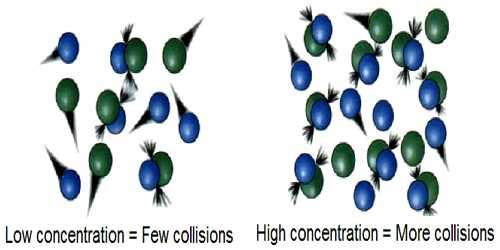Chemistry

# Collision Theory of Reaction RatesCollision Theory of Reaction Rates

In trying to understand the effect of temperature on the rate of reaction it should be recognized that a chemical reaction is only a rearrangement of chemical bonds among the atoms in the reacting system. For such a rearrangement to be effective the reacting molecules must come in contact or a collision between the molecules must take place. Collision theory used to predict the rates of chemical reactions, particularly for gases. Collision theory used to forecast the rates of chemical reactions, mainly for gases. This theory is based on the hypothesis that for a reaction to happen it is essential for the reacting species (atoms or molecules) to come together or collide with one another.

Arrhenius argued that if collision between reactant molecules is a necessary condition for reaction, the rate of reaction should be equal to the frequency of collisions. Calculations, however, show that the rates of most reactions are much smaller than the frequency of collisions. Again, the increase of temperature by 100C results in only a 2% — 3% increase in the frequency of collisions, but the reaction rate increases 200% to 300%. This means that all collisions between reacting molecules do not lead to the reaction.

The question one may ask is “which collisions lead to the reaction?” To answer this question Arrhenius suggested that only ‘active’ molecules can take part in a reaction. These ‘active’ molecules have energies in excess of those possessed by the normal molecules. The minimum extra energy per mole in excess of the average energy which enables the molecules to undergo reaction was termed the activation energy, Ea.

The number of molecules having energies larger than a value Ea may be obtained from the Maxwell-Boltzmann distribution equation, e.g.,

[Number of molecules having energy larger than Ea / Total number of molecules]

= e-E/RT